Next: 1.5 Functions Up: 1. Notation, Undefined Concepts Previous: 1.3 Equality   Index

# 1.4 More Sets

1.44   Definition (Proposition Form.) Let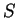be a set. A proposition formonis a rule that assigns to each elementofa unique proposition, denoted by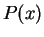.

1.45   Examples. Let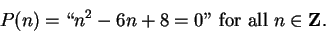Thenis a proposition form on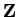.is false, and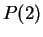is true. Note thatis neither true nor false. A proposition form is not a proposition.

Let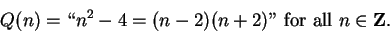(1.46)

Then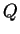is a propostion form, andis true for all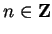. Note thatis not a proposition, but if(1.47)

thenis a proposition andis true. Make sure that you see the difference between the right sides of (1.46) and (1.47). The placement of the quotation marks is crucial. When I define a proposition I will often enclose it in quotation marks, to prevent ambiguity. Without the quotation marks, I would not be able to distinguish between the right sides of (1.46) and (1.47). If I see a statement like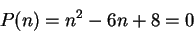without quotation marks, I immediately think this is a statement of the form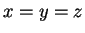and conclude that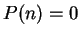.

1.48   Notation. Letbe a set, and letbe a proposition form on. Then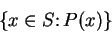(1.49)

denotes the set of all objectsinsuch thatis true. (Read (1.49) as the set of allinsuch that".)

1.50   Examples.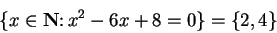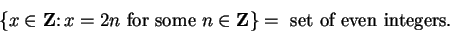Variations on this notation are common. For example,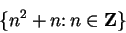represents the set of all numbers of the form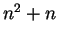where.

1.51   Definition (Union, intersection, difference.) Let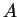be a set, let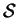be the set of all subsets of, and let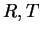be elements of. We define the intersectionofand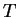by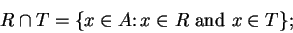we define the union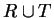ofandby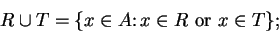and we define the difference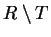by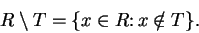1.52   Examples. If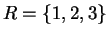and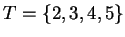, then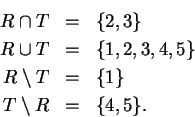1.53   Definition (Ordered pairs and triples.) Let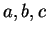be objects (not necessarily all different). The ordered pairis a set-like combination ofand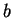into a single object, in whichis designated as the first element andis designated as the second element. The ordered triple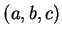is a similar construction havingfor its first element,for its second element andfor its third element. Two ordered pairs (triples) are equal if and only if they have the same first elements, the same second elements, (and the same third elements). Thus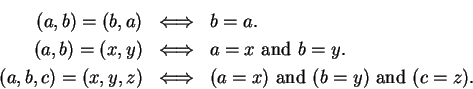1.54   Warning. Ordered pairs should not be confused with sets.1.55   Definition (Cartesian product,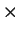.) Ifand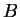are sets, we define the set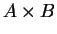by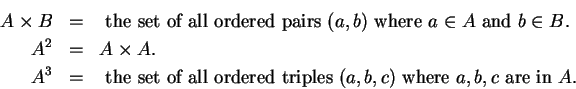is called the Cartesian Product ofand.

1.56   Example. Ifis the set of real numbers, then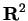is the set of all ordered pairs of real numbers. You are familiar with the fact that ordered pairs of real numbers can be represented as points in the plane, so you can think ofor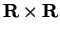as being the points in the plane.Next: 1.5 Functions Up: 1. Notation, Undefined Concepts Previous: 1.3 Equality   Index